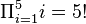# Pi (letter) facts for kids

Kids Encyclopedia Facts
 Quick facts for kidsGreek alphabet Other lettersΑα Alpha Νν Nu Ββ Beta Ξξ Xi Γγ Gamma Οο Omicron Δδ Delta Ππ Pi Εε Epsilon Ρρ Rho Ζζ Zeta Σσ Sigma Ηη Eta Ττ Tau Θθ Theta Υυ Upsilon Ιι Iota Φφ Phi Κκ Kappa Χχ Chi Λλ Lambda Ψψ Psi Μμ Mu Ωω Omega Ϝϝ Digamma Ϟϟ Koppa Ϛϛ Stigma Ϡϡ Sampi Ͱͱ Heta Ϸϸ Sho Ϻϻ San

Pi (uppercase/lowercase Π π), is the letter of the Greek alphabet, used to represent the "p" sound in Ancient and Modern Greek. In the system of Greek numerals, it has a value of 80. Letters that came from it include the Roman P and Cyrillic П.

In mathematics, the uppercase pi is used for the Pi product operator (for example.$\Pi_{i=1}^5 i = 5!$), similar to the way summation is used. The lowercase pi is used as the mathematical constant pi, also known as Archimedes's constant.

## Related pagesPi (letter) Facts for Kids. Kiddle Encyclopedia.